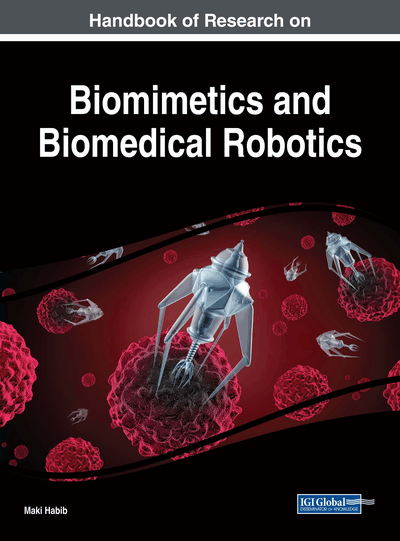# Discrimination of Dual-Arm Motions Using a Joint Posterior Probability Neural Network for Human-Robot Interfaces

Taro Shibanoki (Ibaraki University, Japan) and Toshio Tsuji (Hiroshima University, Japan)
DOI: 10.4018/978-1-5225-2993-4.ch015

## Abstract

This chapter describes a novel dual-arm motion discrimination method that combines posterior probabilities estimated independently for left and right arm movements, and its application to control a robotic manipulator. The proposed method estimates the posterior probability of each single-arm motion through learning using recurrent probabilistic neural networks. The posterior probabilities output from the networks are then combined based on motion dependency between arms, making it possible to calculate a joint posterior probability of dual-arm motions. With this method, all the dual-arm motions consisting of each single-arm motion can be discriminated through leaning of single-arm motions only. In the experiments performed, the proposed method was applied to the discrimination of up to 50 dual-arm motions. The results showed that the method enables relatively high discrimination performance. In addition, the possibility of applying the proposed method for a human-robot interface was confirmed through operation experiments for the robotic manipulator using dual-arm motions.
Chapter Preview
Top

## Background

Many researchers have tried to accurately measure and discriminate the biological signals generated by gestures using various types of sensors and discriminators. The results clearly indicate that the gestures can be used for purposes such as automatic sign-language recognition, and operation of machine control interfaces.

Gesture discrimination studies investigate either static gestures or dynamic gestures. The former are based on hand/arm shapes, and the latter involve time-series patterns of hand/arm motion. In the area of static gestures, a number of studies using a probabilistic neural network (PNN), hidden Markov model (HMM), etc. have been investigated to discriminate several hand shapes (such as the “V for victory” gesture) (Bowden et al., 2002, Bailador, 2007, Okamoto et al., 2008). These techniques can be used to robotic manipulator operations (Brethes et al., 2004, Raheja et al., 2010, Habib, 2011, Devine et al., 2016, Fall et al., 2017). Additionally, Bu et al. proposed a motion discrimination method based on the recurrent probabilistic neural network called a recurrent log-linearized Gaussian mixture network (R-LLGMN) for prosthetic control (Bu et al., 2003, Tsuji et al., 2003, 2006). The generic NN has some drawbacks such as a large-scale network structure and many learning iterations, so that some studies have investigated to integrate domain/task specific knowledge into the architecture of NN (Bridle, 1989, Specht, 1990, Richard and Lippmann, 1991, Caelli et al., 1993). The proposed method integrated hidden Markov model (HMM) and Gaussian mixture model (GMM) into the architecture of NN, and achieves high discrimination performance, even with non-stationary EMG signals during continuous motion. In dynamic gesture discrimination, Liu et al. applied a template-matching method to classify gestures based on acceleration signals relating to three dimensions, then discriminated eight dynamic gestures with an accuracy level of 98% and developed a gesture-based interface for a cellular phone. Solís et al. also investigated the discrimination of 21 gestures with 93% accuracy using an artificial neural network based on RGB images while gestures were being made. However, these studies focused exclusively on single-arm motion discrimination (Liu et al., 2009, Huang et al., 2015, Solís et al., 2016). As gestures include both left- and right-arm motion among their characteristics, it is necessary to discuss a discrimination method for dual-arm motion in addition to considering single-arm motion.

## Key Terms in this Chapter

Human-Robot Interface: A controlling method and controller itself for operation of robots. Biological signals such as electromyograms, electroencephalograms, acceleration signals are often used as a control input.

Joint Probability Neural Network (J-PNN): One of the artificial recurrent probabilistic neural networks (ARPNN) consisting of two ARPNNs incorporated a hidden Markov model and a Gaussian mixture model.

Recurrent Probabilistic Neural Network: An artificial recurrent neural network that can estimate the probability density function of a given set of time series data. It is used for classification problems because it can compute nonlinear decision boundaries between each discrimination class.

Joint Posterior Probability: A simultaneous revised probability that can be calculated using J-PNN combining independently estimated posterior probabilities for each arm motion based on entropy.

Motion Dependency: A property that an arm movement is followed by the other ones performing dual-arm motions (e.g., asymmetric motions).

Gesture Recognition: A technique of recognizing bodily movements (such as sign languages) using biological signals.

Motion Acceleration Signal (MAC): A record of velocity changes occurred corresponding to bodily movement. Motion acceleration (MAC) and mechanomyogram (MMG) components can be separated from raw acceleration signals using appropriate digital filter because of the difference of frequency band.

## Complete Chapter List

Search this Book:
Reset